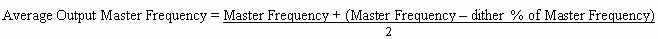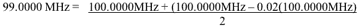# How to 'Center' a Down-Dithering EconOscillator

### Abstract

This application note describes how to use a down-dithering EconOscillator™ in an application that requires a centered spread-spectrum output frequency. In most instances, the amount of down-dither and/or output frequency is adjustable. Nonetheless, calculating the appropriate register values to achieve centered operation from a down-dithering EconOscillator is not always straightforward. An example shows how to compensate for the down-dither effects on an average output frequency.

### Introduction to the Problem

Some EconOscillators have a selectable spread-spectrum (dithered) output. When dithering is enabled, not only does the frequency vary with time, but the average output frequency is also lowered. This unexpected reduction in the average output frequency is undesirable in some applications. In other applications this frequency reduction is, in fact, useful. This is true because the output frequency never exceeds the maximum frequency set by the master oscillator, and still meets stringent spectral-emission requirements.

In the dithering EconOscillator, a triangle-wave generator injects an offset element below the master oscillator's control signal. This results in a continuously shifting output frequency, which is no longer centered (i.e., a 'mean' output frequency) at the programmed master oscillator's frequency. This means that the triangle-wave only reduces the output frequency. Figure 1 illustrates this process.Some applications for dithering EconOscillators may not be limited by the requirement for an absolute maximum frequency. In these cases wherever dither is still required, the EconOscillator can be programmed so that the average output frequency is precisely centered at the desired value. See Figure 2.### Solution to the Problem

The solution to this problem is actually quit simple. To achieve the desired average frequency, recalculate the master frequency to a higher value. Recalculating the master frequency higher by 1/2 the dither value will compensate the average output frequency when dither is enabled.If, for example, a DS1086 is programmed with the factory default offset (OS), a DAC register of 784dec, and dither disabled, the average output frequency will be 100.0000MHz. However, if dither is enabled at 2%, the average output frequency will be shifted down. The new average frequency will be:The output will have an average frequency of 99.0000MHz, a 2% dither, and sweep between 98.0000MHz and 100.0000MHz. If the application requires the center frequency to remain at 100.0000MHz, then a different DAC register setting must be chosen.

In applications requiring dither at 2% and an average output frequency of 100.000MHz, simply select a new DAC register value to shift the master oscillator frequency up. In this latter case choose a DAC register of 885dec.### Conclusion

This application note describes how to calculate a master oscillator value to realize a centered output frequency when using a down-dithering EconOscillator. By recalculating and programming the EconOscillator's register settings, the output frequency and dither can be precisely adjusted to achieve desired results.

Questions/comments/suggestions concerning this application note can be sent to Analog document feedbacks.